Author: Oscar Cronquist Article last updated on January 15, 2019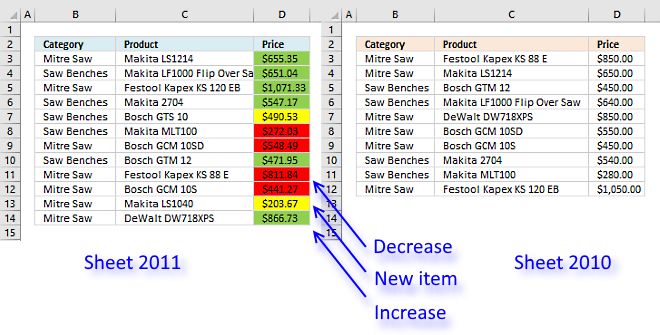Today I am going to show you how to quickly compare two tables using Conditional Formatting (CF). I am going to compare two price lists from the year 2010 and year 2011.

I have two types of data set layouts I want to share a solution for, basic dataset layout shown above and a two-index layout.

To make things more authentic in my examples, products in price list 2011 are not sorted in the same way as 2010. New products are also introduced.

It is quite common that price lists are huge and a total mess. Excel is the perfect tool for finding the differences between datasets.

A requirement for these conditional formatting formulas to work, is that column and row headers have identical spelling.

The same letter capitalization is not required.

### Basic dataset layout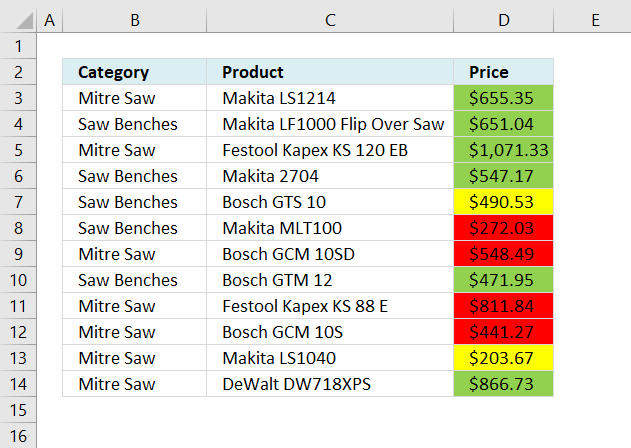I have two worksheets, in this example, named 2010 and 2011. They both contain a category, product, and a price column.

 Color Description Yellow A new item in the list. Green Price increase. Red Price decrease.

There are three different CF formulas applied to cell range D3:D14, each coloring a cell based on a condition.

Two or more CF formulas can't color cell at the same time, the logical expressions I built can't all be true at the same time.

#### Red Conditional Formatting formula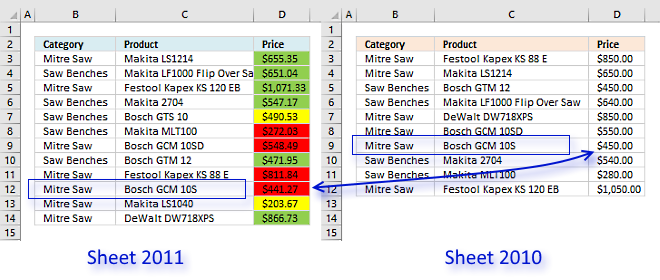The red CF formula compares the value in column D based on category value and product value with the corresponding product, category and price in worksheet 2010. If the value in column D is smaller the cell is highlighted red.

=\$D3<INDEX(2010'!\$D\$3:\$D\$12, MATCH(1,COUNTIFS(\$B3, 2010'!\$B\$3:\$B\$12, \$C3, 2010'!\$C\$3:\$C\$12), 0))

The COUNTIFS function returns an array that indicates the position of the corresponding price. The following explanation is for cell D12, see picture above.

COUNTIFS(\$B12, 2010'!\$B\$3:\$B\$12, \$C12, 2010'!\$C\$3:\$C\$12) returns {0; 0; 0; 0; 0; 0; 1; 0; 0; 0}.

The MATCH function returns the position of a given value in the array.

MATCH(1,COUNTIFS(\$B12, 2010'!\$B\$3:\$B\$12, \$C12, 2010'!\$C\$3:\$C\$12), 0)

becomes

MATCH(1,{0; 0; 0; 0; 0; 0; 1; 0; 0; 0}, 0) and returns 7. Now we know where the value we are looking for is.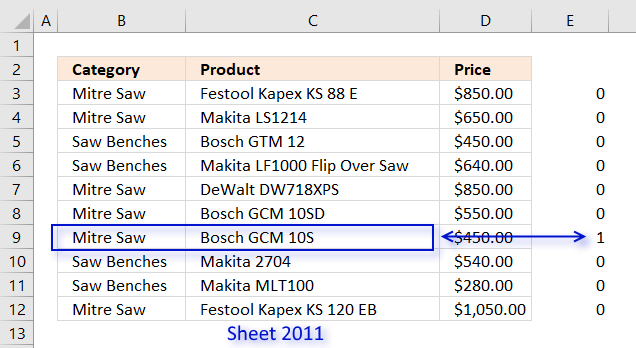\$D12<INDEX(2010'!\$D\$3:\$D\$12, MATCH(1,COUNTIFS(\$B12, 2010'!\$B\$3:\$B\$12, \$C12, 2010'!\$C\$3:\$C\$12), 0))

becomes

\$D12<INDEX(2010'!\$D\$3:\$D\$12, 7)

becomes

\$441.27<\$450 and returns TRUE.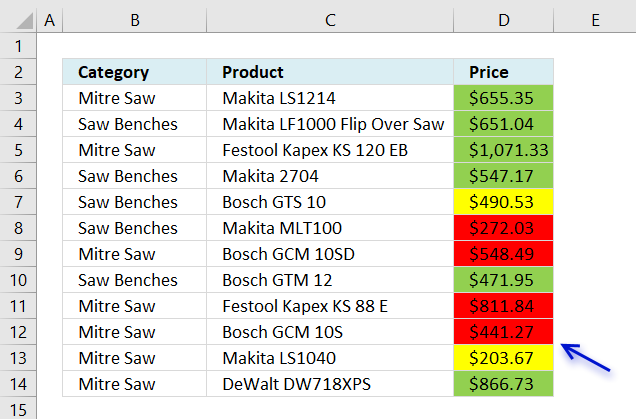Cell D12 is highlighted red.

#### Green Conditional Formatting formula

\$D3>INDEX(2010'!\$D\$3:\$D\$12, MATCH(1, COUNTIFS(\$B3, 2010'!B\$\$3:\$B\$12, \$C3,2010'!\$C\$3:\$C\$12), 0))

The green CF formula is similar to the red CF formula except that the cell is highlighted green if the value in column D is larger than the corresponding value in worksheet 2010.

The only difference between the red and green CF formulas is the larger than and smaller than sign.

#### Yellow Conditional Formatting formula

=COUNTIFS('2010'!B3:B12,\$B3,'2010'!C3:C12,\$C3)=0

The yellow CF formula checks if the category and product is not found in worksheet 2010, if TRUE the cell is highlighted yellow.

Compare-pricelists_newv2.xlsx

### Two index table

Here are the two tables together on the same worksheet.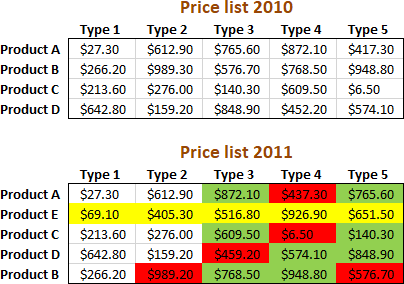As you can see "product E" is new for 2011 (highlighted yellow), "product A" type 4 has a lower price than the previous year 2010 (highlighted red), etc.

The colors make it very easy to spot differences.

#### New values compared to last year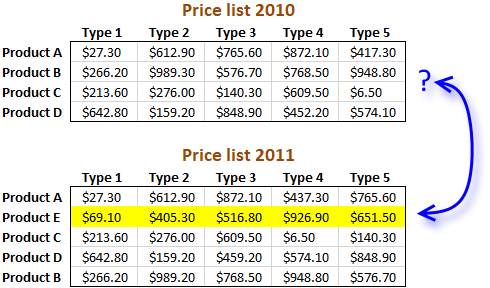The following CF formula highlights entire row yellow if it finds a new product name.

Conditional formatting formula:

=ISERROR(INDEX(\$C\$4:\$G\$7, MATCH(\$B11, \$B\$4:\$B\$7, 0), MATCH(C\$10, \$C\$3:\$G\$3, 0))<>C11)

It compares the product and type columns between the tables and if a value is not found the CF formula highlights the entire row yellow.

#### Find lower prices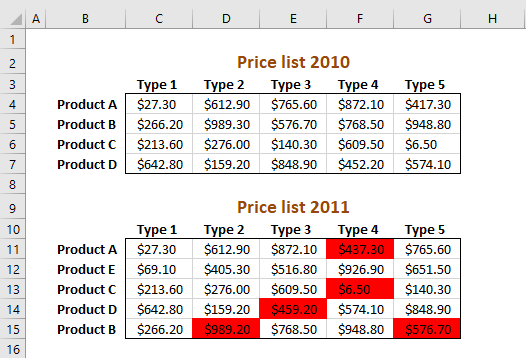Conditional formatting formula:

=INDEX(\$C\$4:\$G\$7, MATCH(\$B11, \$B\$4:\$B\$7, 0), MATCH(C\$10, \$C\$3:\$G\$3, 0))>C11

Cells are formatted red if the price is lower than the price in the other table.

#### Find higher prices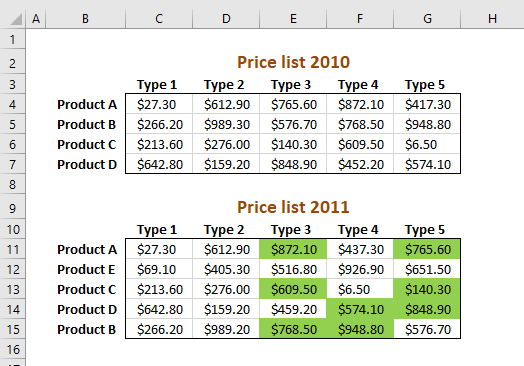Conditional formatting formula:

=INDEX(\$C\$4:\$G\$7, MATCH(\$B11, \$B\$4:\$B\$7, 0), MATCH(C\$10, \$C\$3:\$G\$3, 0))<C11

Cells are formatted green if the price is higher than the price in the other table.

#### How to apply conditional formatting formula

1. Select cells C11:G15
2. Click "Home" tab
3. Click "Conditional Formatting" button
4. Click "New Rule.."
5. Click "Use a formula to determine which cells to format"
6. Copy and paste conditional formatting formula in "Format values where this formula is TRUE" window.
7. Click "Format.." button
8. Click "Fill" tab
9. Select a color for highlighted cells.
10. Click "Ok"
11. Click "Ok"
12. Click "Ok"

#### Explaining find lower prices conditional formatting formula in cell C11

You can follow along, copy the CF formula and paste it in a cell.

Go to tab "Formula" on the ribbon and then click on "Evaluate Formula" button.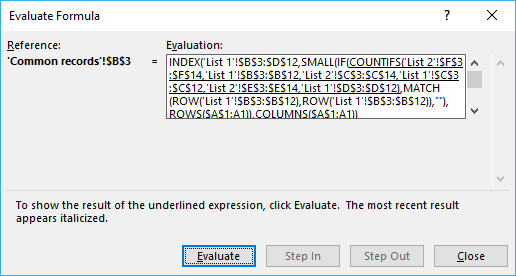Click on the "Evaluate" button to move to the next step in the calculations.

Step 1 - Find relative position of current row header in previous pricelist

=INDEX(\$C\$4:\$G\$7, MATCH(\$B11, \$B\$4:\$B\$7, 0), MATCH(C\$10, \$C\$3:\$G\$3, 0))<C11

The MATCH function returns the relative position of an item in an array that matches a specified value.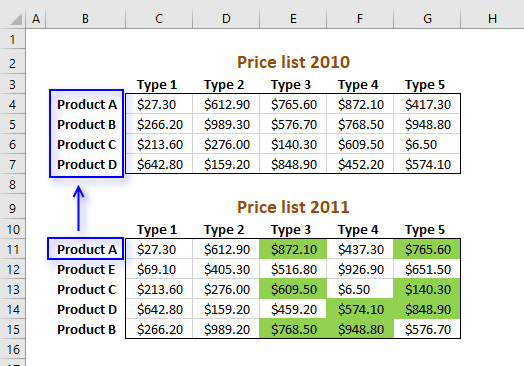MATCH(\$B11, \$B\$4:\$B\$7, 0)

becomes

MATCH("Product A", {"Product A";"Product B";"Product C";"Product D"}, 0)

and returns 1.

Step 2 - Find relative position of current column header in previous price list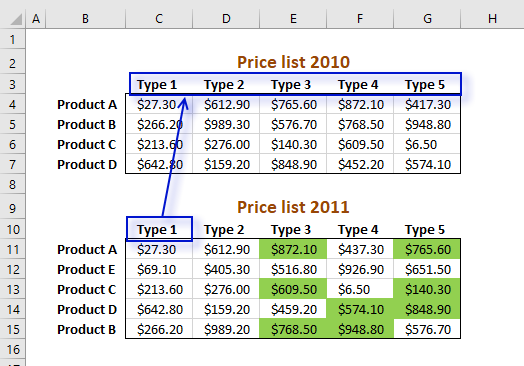=INDEX(\$C\$4:\$G\$7, MATCH(\$B11, \$B\$4:\$B\$7, 0), MATCH(C\$10, \$C\$3:\$G\$3, 0))<C11

MATCH(C\$10, \$C\$3:\$G\$3, 0)

becomes

MATCH("Model 1", {"Model 1", "Model 2", "Model 3", "Model 4", "Model 5"}, 0)

returns 1. Type 1 is found in position 1 in cell range C3:G3.

Step 3 - Return a value of the cell at the intersection of a particular row and column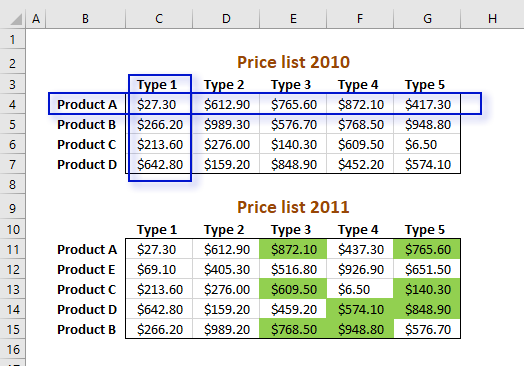=INDEX(\$C\$4:\$G\$7, MATCH(\$B11, \$B\$4:\$B\$7, 0), MATCH(C\$10, \$C\$3:\$G\$3, 0))<C11

becomes

=INDEX(\$C\$4:\$G\$7, 1, 1)

becomes

=INDEX({27,3, 612,9, 765,6, 872,1, 417,3;266,2, 989,3, 576,7, 768,5, 948,8;213,6, 276, 140,3, 609,5, 6,5;642,8, 159,2, 848,9, 452,2, 574,1}, 1, 1)

returns 27,3. The picture above shows how the formula finds the value at the intersection of a given row and column number.

Step 4 - Compare returned value to current value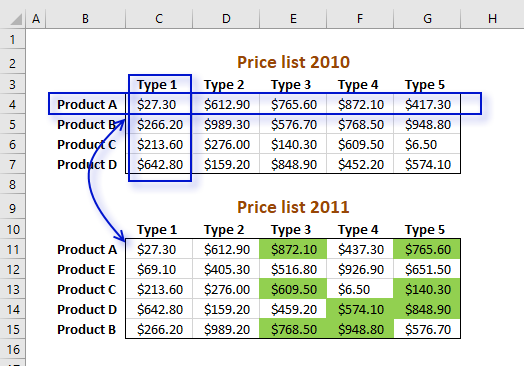=INDEX(\$C\$4:\$G\$7, MATCH(\$B11, \$B\$4:\$B\$7, 0), MATCH(C\$10, \$C\$3:\$G\$3, 0))<C11

becomes

27,3>C11

becomes

27,3>27,3

returns FALSE. Cell C11 is not highlighted green.